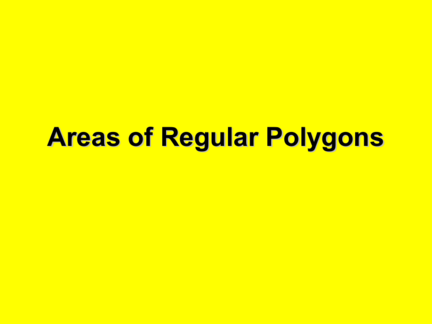# Area of Regular PolygonsContributed by:This pdf contains the area of a regular polygon which is one-half the product of its apothem and its perimeter. Often the formula is written like this: Area=1/2(ap), where a denotes the length of an apothem, and p denotes the perimeter
1. Areas of Regular Polygons
2. Lesson Focus
The focus of this lesson is on applying the
formula for finding the area of a regular
3. Basic Terms
Center of a Regular Polygon
the center of the circumscribed circle
the distance from the center to a vertex
Central Angle of a Regular Polygon
an angle formed by two radii drawn to
consecutive vertices
Apothem of a Regular Polygon
the (perpendicular) distance from the center
of a regular polygon to a side
4. Basic Terms
5. Theorem 11-11
The area of a regular polygon is equal to
half the product of the apothem and the
6. Area of a regular polygon
The area of a regular polygon is:
A = ½ Pa
Area
Perimeter
apothem
7. The center of circle A is:
A
B The center of pentagon
BCDEF is:
A
F C A radius of circle A is:
A AF
BCDEF is:
G AF
E D An apothem of pentagon
BCDEF is:
AG
8. Area of a Regular Polygon
• The area of a regular n-gon with side lengths (s)
is half the product of the apothem (a) and the
perimeter (P), so
The number of congruent
triangles formed will be
A = ½ aP, or A = ½ a • ns. the same as the number of
sides of the polygon.
NOTE: In a regular polygon, the length of each
side is the same. If this length is (s), and there
are (n) sides, then the perimeter P of the
polygon is n • s, or P = ns
9. More . . .
• A central angle of a regular polygon is an
angle whose vertex is the center and
whose sides contain two consecutive
vertices of the polygon. You can divide
360° by the number of sides to find the
measure of each central angle of the
polygon.
• 360/n = central angle
10. Areas of Regular Polygons
Center of a regular polygon: center of the circumscribed circle.
Radius: distance from the center to a vertex.
Apothem: Perpendicular distance from the center to a side.
Example 1: Find the measure of each numbered angle. 3
2
360/5 = 72 ½ (72) = 36 L2 = 36 1 •
L1 = 72 L3 = 54
Area of a regular polygon: A = ½ a p where a is the apothem and p is the perimeter.
Example 2: Find the area of a regular decagon with a 12.3 in apothem and 8 in sides.
Perimeter: 80 in A = ½ • 12.3 • 80 A = 492 in2
Example 3: Find the area.
10 mm
A=½ap p = 60 mm •
LL = √3 • 5 = 8.66 a
5 mm
A = ½ • 8.66 • 60 A = 259.8 mm2
11. • But what if we are not given any angles.
12. Ex: A regular octagon has a radius
of 4 in. Find its area.
First, we have to find the
67.5
o
x
apothem length.
a x
4 sin 67.5  cos 67.5 
4 4
a
3.7 4cos67.5 = x
4sin67.5 = a
135o 3.7 = a 1.53 = x
Now, the side length.
Side length=2(1.53)=3.06
A = ½ Pa = ½ (24.48)(3.7) = 45.288 in2
13. Last Definition
Central  of a polygon – an  whose
vertex is the center & whose sides
contain 2 consecutive vertices of the
polygon.
Y is a central .
Measure of a
360
central  is: n Y
Ex: Find mY.
72o
14. Check out!
ygonregulararea.html